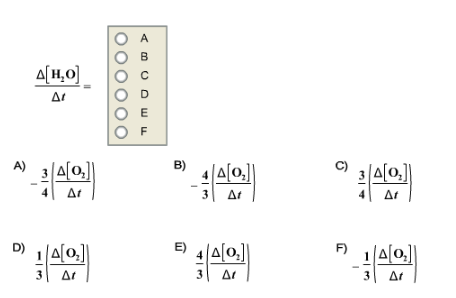# Problem: Given the following balanced chemical equation 3 O2 + 2 CH3OH → 2 CO2 + 4 H2OHow is the rate of appearance of H2O related to the rate of disappearance of O2?

###### FREE Expert Solution

Recall that for a reaction aA  bB, the rate of a reaction is given by:

$\overline{){\mathbf{Rate}}{\mathbf{=}}{\mathbf{-}}\frac{\mathbf{1}}{\mathbf{a}}\frac{\mathbf{\Delta }\mathbf{\left[}\mathbf{A}\mathbf{\right]}}{\mathbf{\Delta t}}{\mathbf{=}}\frac{\mathbf{1}}{\mathbf{b}}\frac{\mathbf{\Delta }\mathbf{\left[}\mathbf{B}\mathbf{\right]}}{\mathbf{\Delta t}}}$

where:

Δ[A] = change in concentration of reactants or products (in mol/L or M), [A]final – [A]initial

Δt = change in time, tfinal – tinitial

Reaction: 3 O2 + 2 CH3OH → 2 CO2 + 4 H2O

H2O is a product, the rate with respect to H2O is positive (+) since we’re gaining products.

O2 is a reactant, the rate with respect to O2 is negative (–) since we’re losing reactants.

86% (321 ratings)###### Problem Details

Given the following balanced chemical equation

3 O2 + 2 CH3OH → 2 CO2 + 4 H2O

How is the rate of appearance of H2O related to the rate of disappearance of O2Frequently Asked Questions

What scientific concept do you need to know in order to solve this problem?

Our tutors have indicated that to solve this problem you will need to apply the Average Rate of Reaction concept. You can view video lessons to learn Average Rate of Reaction. Or if you need more Average Rate of Reaction practice, you can also practice Average Rate of Reaction practice problems.

What professor is this problem relevant for?

Based on our data, we think this problem is relevant for Professor Hogan's class at LSU.